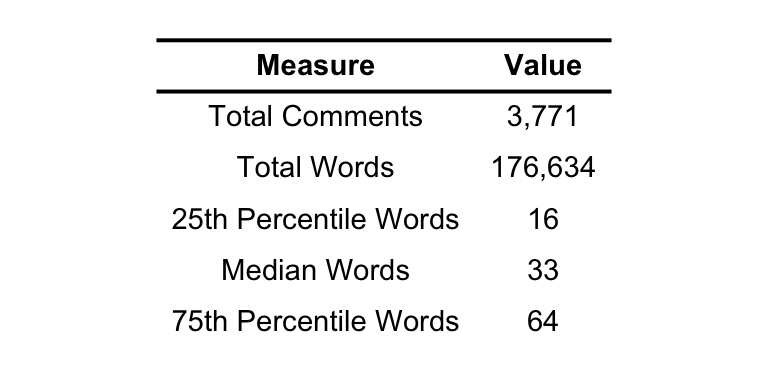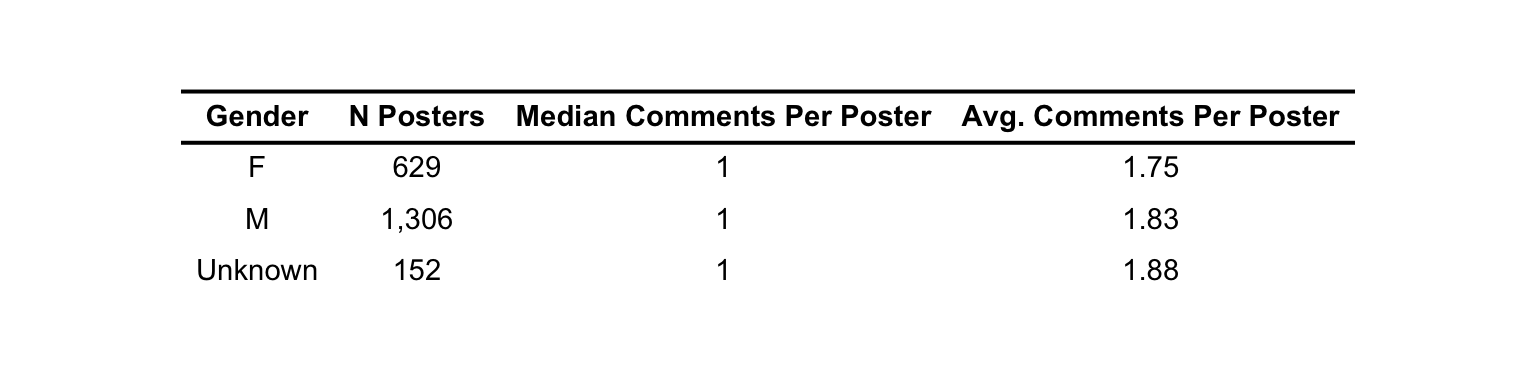This is an RMarkdown document with code to analyze the online comments on the Wall Street Journal opinion piece ‘Is There a Doctor in the White House? Not if You Need an MD.’

## Setup

library(tidyverse)
library(gender)
library(cleanNLP)
library(glmnet)
library(ggpubr)
library(grid)
library(gridExtra)
library(wesanderson)

# Proxy for gender using SSA recrods for individuals born from 1932 - 2002
dat_in2 <- dat_in %>%
mutate(first_name = tolower(str_extract(name, "([^\\s]+)"))) %>%
mutate(
birth_year_min = "1932",
birth_year_max = "2002")

name_gender_probs <- gender_df(
dat_in2,
name_col = "first_name",
year_col = c("birth_year_min", "birth_year_max"),
method="ssa") %>%
distinct(name, proportion_female)

dat_final <- dat_in2 %>%
left_join(name_gender_probs, by=c("first_name"="name")) %>%
select(name, first_name, prob_name_female=proportion_female, comment)

## Comment Summary 1

# Summary table of the raw comments
dat_final %>%
mutate(words = sapply(strsplit(comment, " "), length)) %>%
summarise(
Total Comments = n(),
Total Words = sum(words),
25th Percentile Words= quantile(words, .25),
Median Words= quantile(words, .5),
75th Percentile Words= quantile(words, .75)
) %>%
gather(key="Measure", value="Value") %>%
mutate(Value = prettyNum(Value, big.mark=",")) %>%
ggtexttable(rows = NULL, theme = ttheme("blank")) %>%
tab_add_hline(at.row = 1:2, row.side = "top", linewidth = 4)## Comment Summary 2

# Many authors comment more than once. Here we make each observation a
# unique author (first name / last name combination)
dat_by_author <- dat_final %>%
group_by(name) %>%
summarise(
first_name = first(first_name),
prob_name_female = first(prob_name_female),
comment = paste(comment, collapse = " "),
# Only use individuals where we are >= 75% sure about their gender
mutate(gender = case_when(
prob_name_female >= 0.75 ~ "F",
prob_name_female <= 0.25 ~ "M",
TRUE ~ "Unknown"
))

dat_by_author %>%
group_by(gender) %>%
summarise(
N Posters = n(),
Median Comments Per Poster = median(num_comments),
Avg. Comments Per Poster = mean(num_comments),
) %>%
mutate(
N Posters = prettyNum(N Posters, big.mark=","),
Avg. Comments Per Poster = round(Avg. Comments Per Poster, 2)) %>%
rename(Gender = gender) %>%
ggtexttable(rows = NULL, theme = ttheme("blank")) %>%
tab_add_hline(at.row = 1:2, row.side = "top", linewidth = 4)## Annotate the Data

# Filter out unknown gender
dat_by_author <- dat_by_author %>%
filter(gender != "Unknown")

# Start up the udpipe init of cleanNLP.
# cleaNLP will tokenize the text data, allowing us to limit
# to certain parts of speech and lemmatise each word.
# See: https://statsmaths.github.io/cleanNLP/
cnlp_init_udpipe()

# Final preperations for the annotation
dat_to_anno <- dat_by_author %>%
rename(text=comment) %>%
mutate(doc_id = 1:n())

# This annotation step takes somewhat long to run
anno <- cnlp_annotate(dat_to_anno)

## Find Marginal Effects

Here, we use a simple Lasso regression model, loosely basing our methodology on Wu, 2018. Specifically, letting $$w_i$$ denote a vector of indicators for whether each of the lemmatised verbs, adjectives, and nouns used by at least 25 commenters is used by commenter $$i$$, we estimate a Lasso linear regression model for the probability that the post is authored by a $$Female$$, as follows:

$$\hat{\beta_{\lambda}} = argmin_{\beta}\sum_i(Female_i - \beta_0-w_i'\beta)^2+\lambda||\beta||_1$$

where $$||\beta||_1 = \sum_{j\geq1}|\beta^j|$$

The marginal effect of word $$k$$ on the probability that an author is $$Female$$ is estimated by $$\hat{\beta_{\lambda}^k}$$, the coefficient on the regressor $$w_i^k$$. We select an optimal tuning parameter $$\lambda^*$$ through 10-fold cross validation. Importantly, Lasso regression’s functional form helps identify words without over-fitting our model. There are 425 words used by at least 25 commenters.

# Set a seed for reproducibility
set.seed(21)

# Filter to Nouns, Adjectives, and Verbs
df <- anno$token %>% left_join(anno$document, by="doc_id") %>%

# Build the term-frequency matrix.
# We use binary (0/1 - does this author use the word?).
# min_df is the minimum share of documents the word must be used in to be used,
# we will set is so that a word needs to be in 25 documents.
min_docs_used <- 25

# Build the term frequency matrix
mat <- cnlp_utils_tf(df, doc_var = "doc_id", "binary", min_df=min_docs_used/max(df$doc_id)) # Make the vector of outcome variables as 0/1 gender <- df %>% distinct(doc_id, gender) %>% mutate(gender_F = as.numeric(gender == "F")) # Use 10-fold cross validation to set the lambda tuning parameter cv <- cv.glmnet(mat, gender$gender_F, alpha = 1, family = "gaussian", nfolds=10)

# Now use that lambda to predict
model <- glmnet(mat, gender$gender_F, alpha = 1, lambda = cv$lambda.min, family = "gaussian")

# Get the betas
beta <- coef(model)[-1]

# Get the non-zero betas
final <- tibble(
word = colnames(mat)[beta != 0],
coef = beta[beta != 0]
) 

## Most Predictive Words

# Most female predictive
female <- final %>%
arrange(desc(coef)) %>%
slice(1:20) %>%
mutate(coef = round(coef, 3)) %>%
rename(Word=word, Marginal Effect=coef)

# Most male predictive
male <- final %>%
arrange(coef) %>%
slice(1:20) %>%
mutate(coef = round(coef, 3)) %>%
rename(Word=word, Marginal Effect=coef)

female_p <- ggtexttable(female, rows = NULL, theme = ttheme("blank")) %>%
tab_add_hline(at.row = 1:2, row.side = "top", linewidth = 4)

male_p <- ggtexttable(male, rows = NULL, theme = ttheme("blank")) %>%
tab_add_hline(at.row = 1:2, row.side = "top", linewidth = 4)

grid.arrange(
female_p + labs(title="\n \n Most Female") +
theme(legend.position = "none", plot.title = element_text(size=13, hjust=0.5)),
male_p +
labs(title = "\n \n Most Male") +
theme(legend.position = "none", plot.title = element_text(size=13, hjust=0.5)),

top = textGrob("Words Most Predictive of Male/Female Commenters",
gp=gpar(fontsize=17)),

nrow = 1)